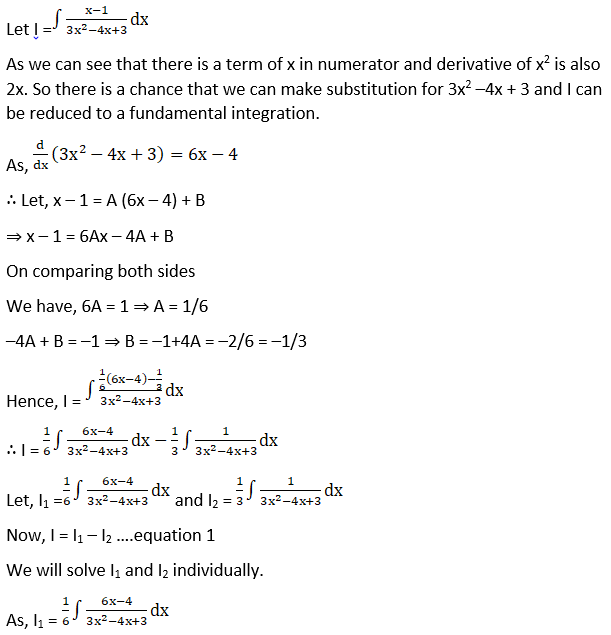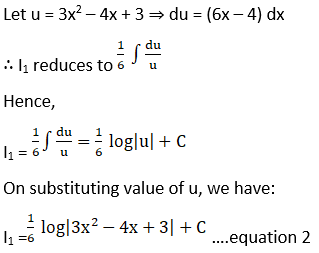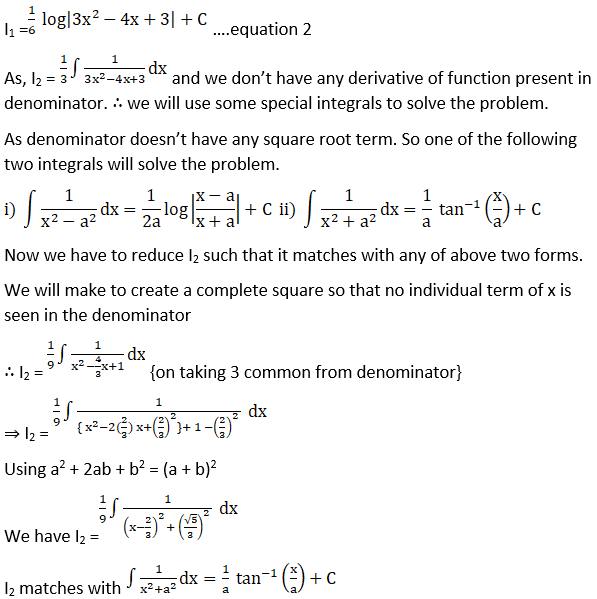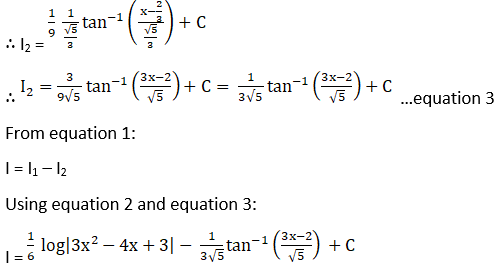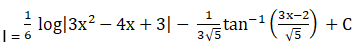# RD Sharma Solutions For Class 12 Maths Exercise 19.19 Chapter 19 Indefinite Integrals

RD Sharma Solutions for Class 12 Maths Exercise 19.19 Chapter 19 Indefinite Integrals is provided here. Students facing any difficulties in understanding concepts of this chapter can refer to the RD Sharma Solutions for Class 12 Maths developed by subject matter experts at BYJU’S. All the solutions are created with an aim to boost confidence for their exam preparations. The resource also improves problem-solving skills of the students, which are essential from an examination standpoint.

The PDF of RD Sharma Solutions for Class 12 Maths Chapter 19 Exercise 19.19 can be accessed from the link given below. This exercise explains how to determine integrals of the form

$$\begin{array}{l}\int \frac{px+q}{ax^{2}+bx+c}\ dx\end{array}$$
with algorithm.

## Download the PDF of RD Sharma Solutions For Class 12 Chapter 19 – Indefinite Integrals Exercise 19.19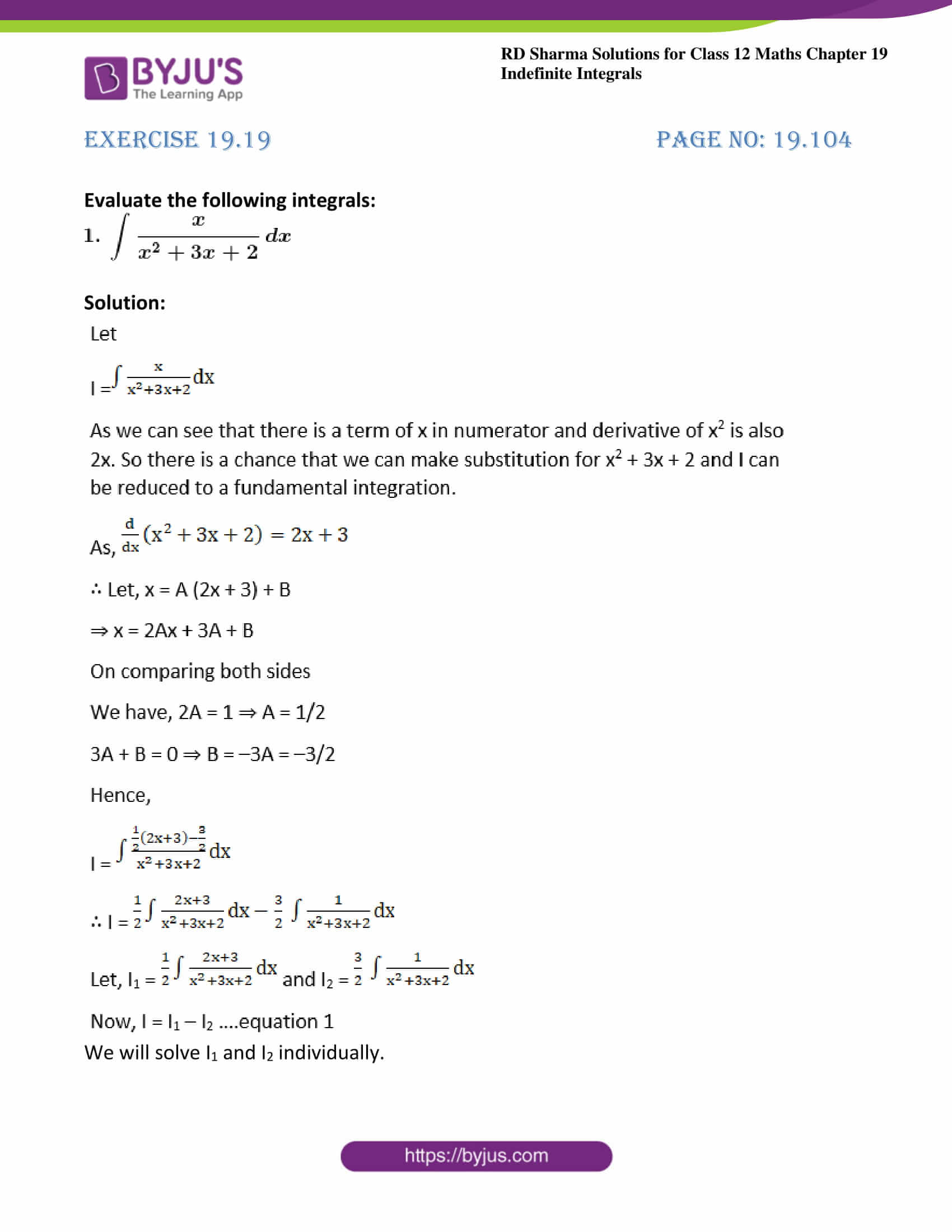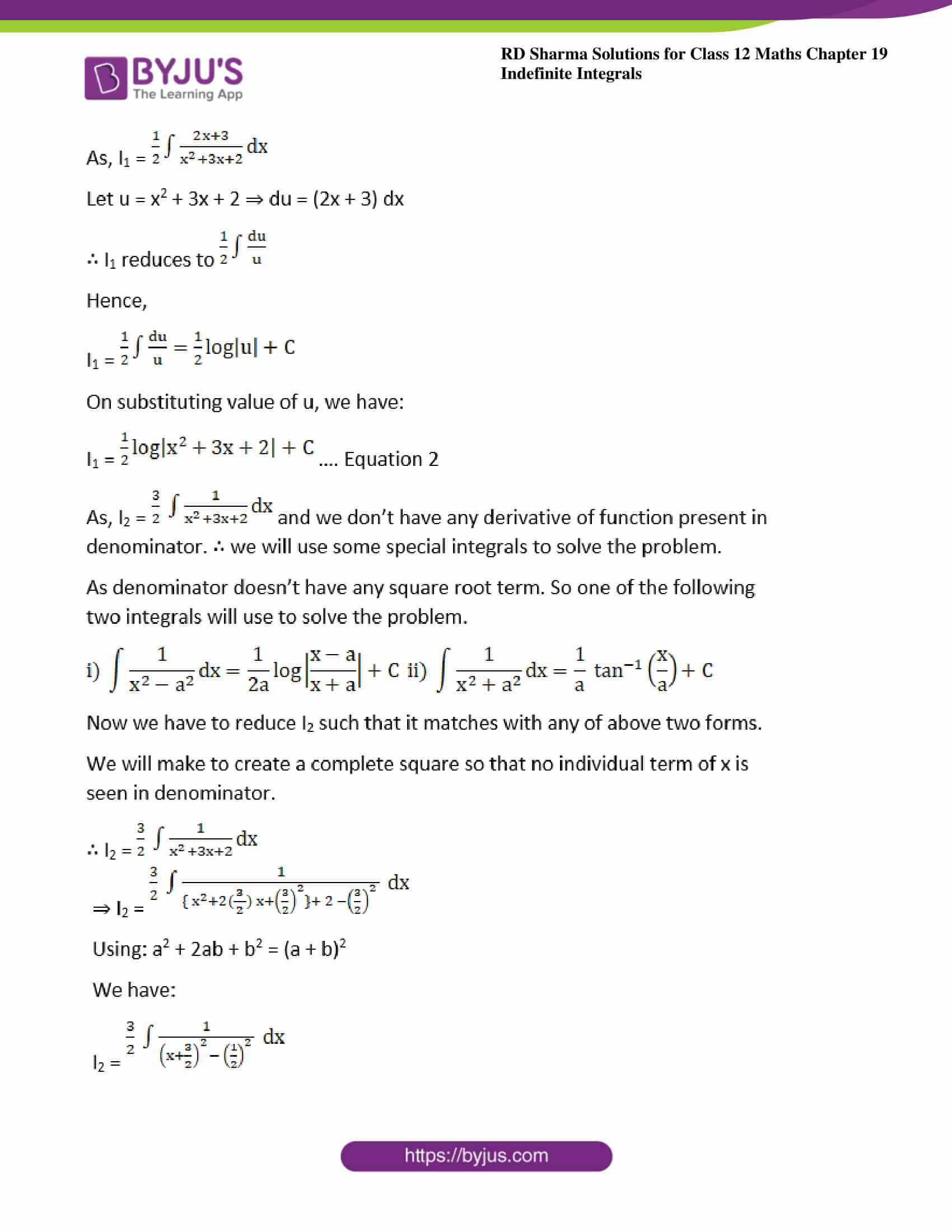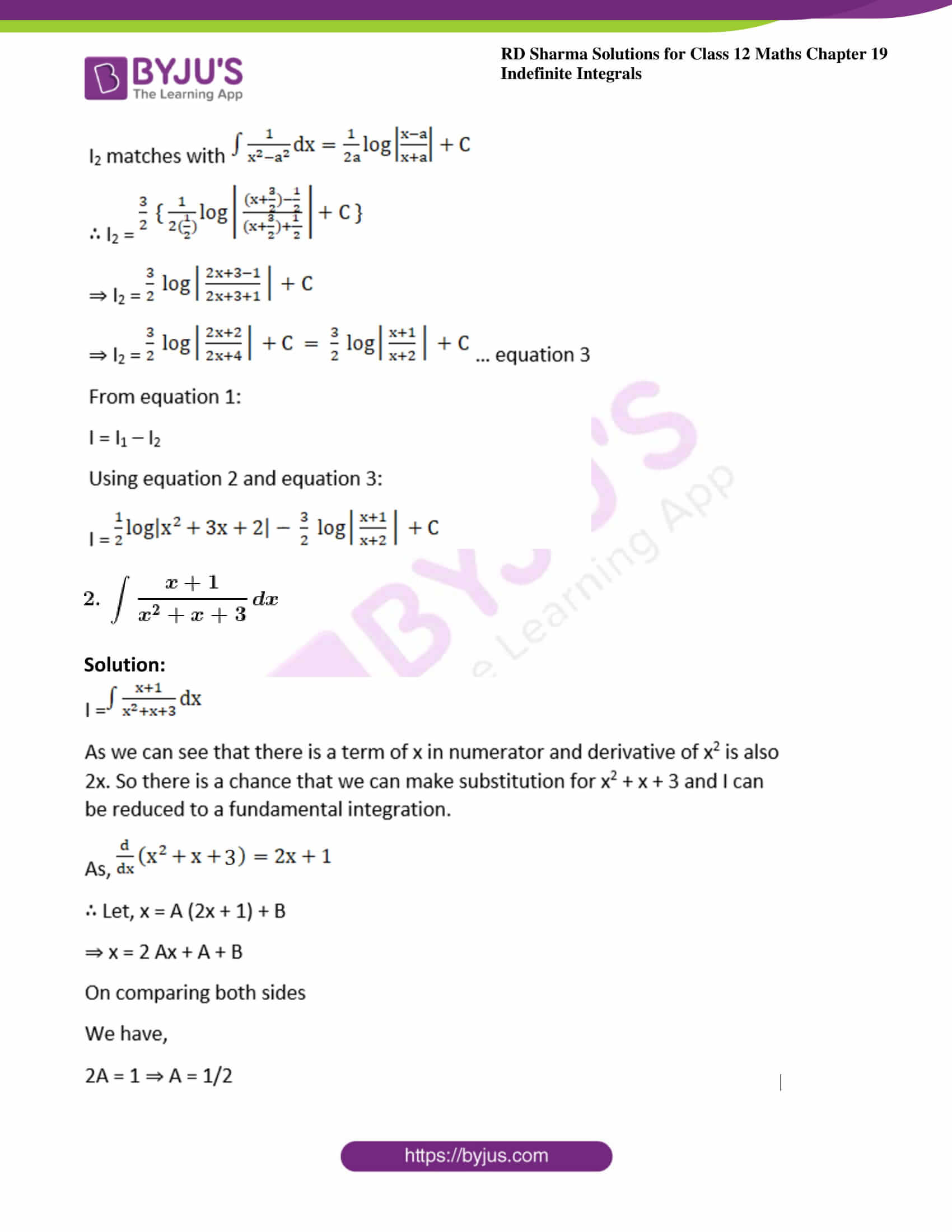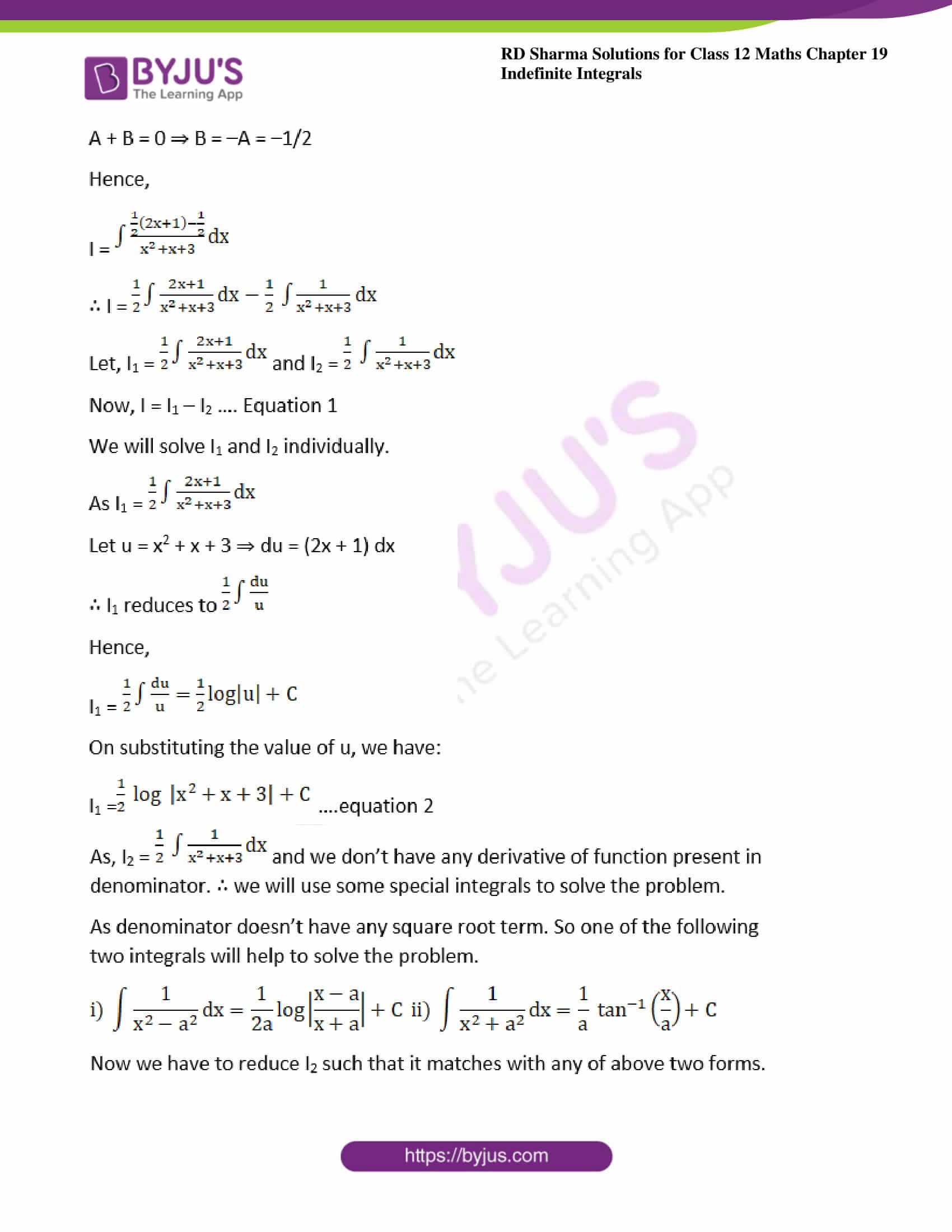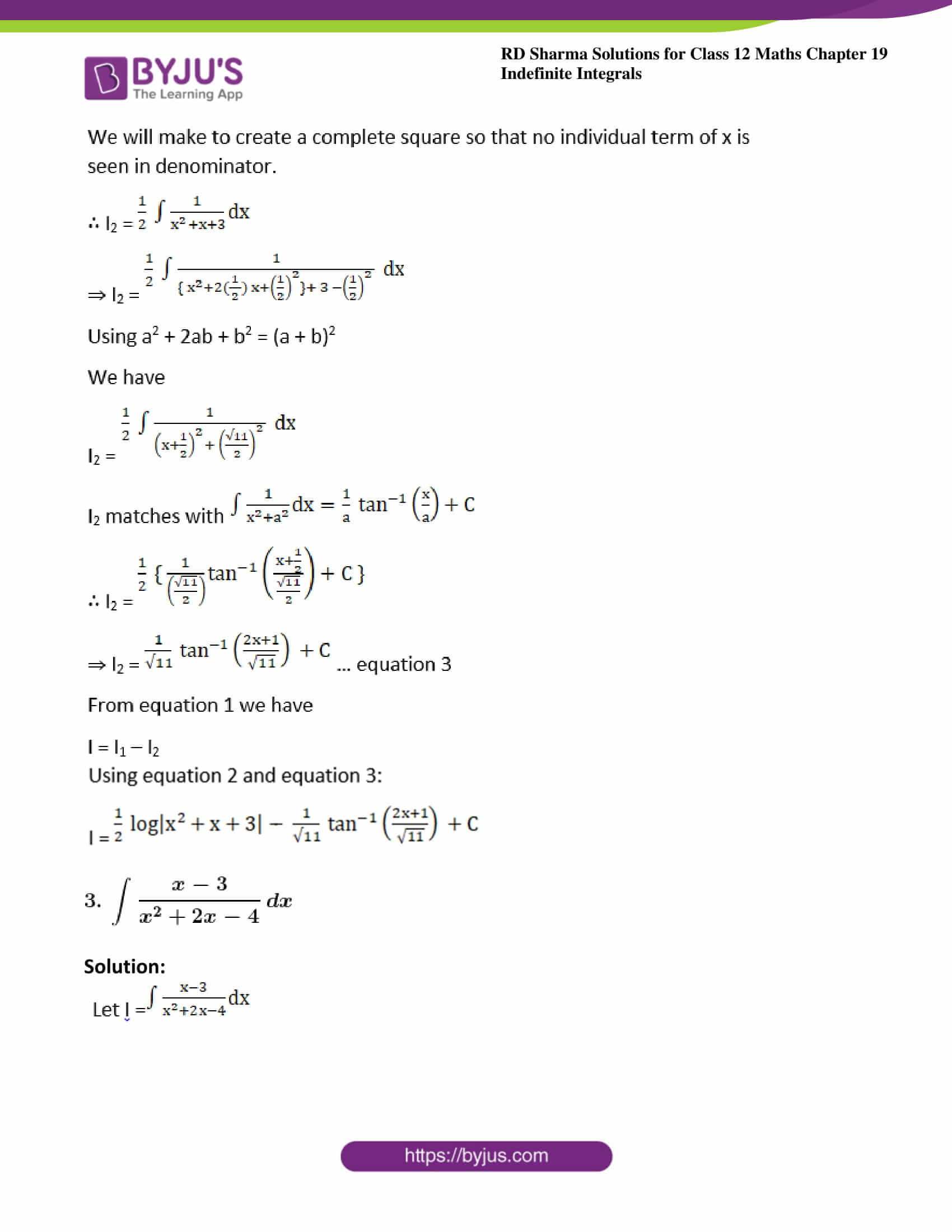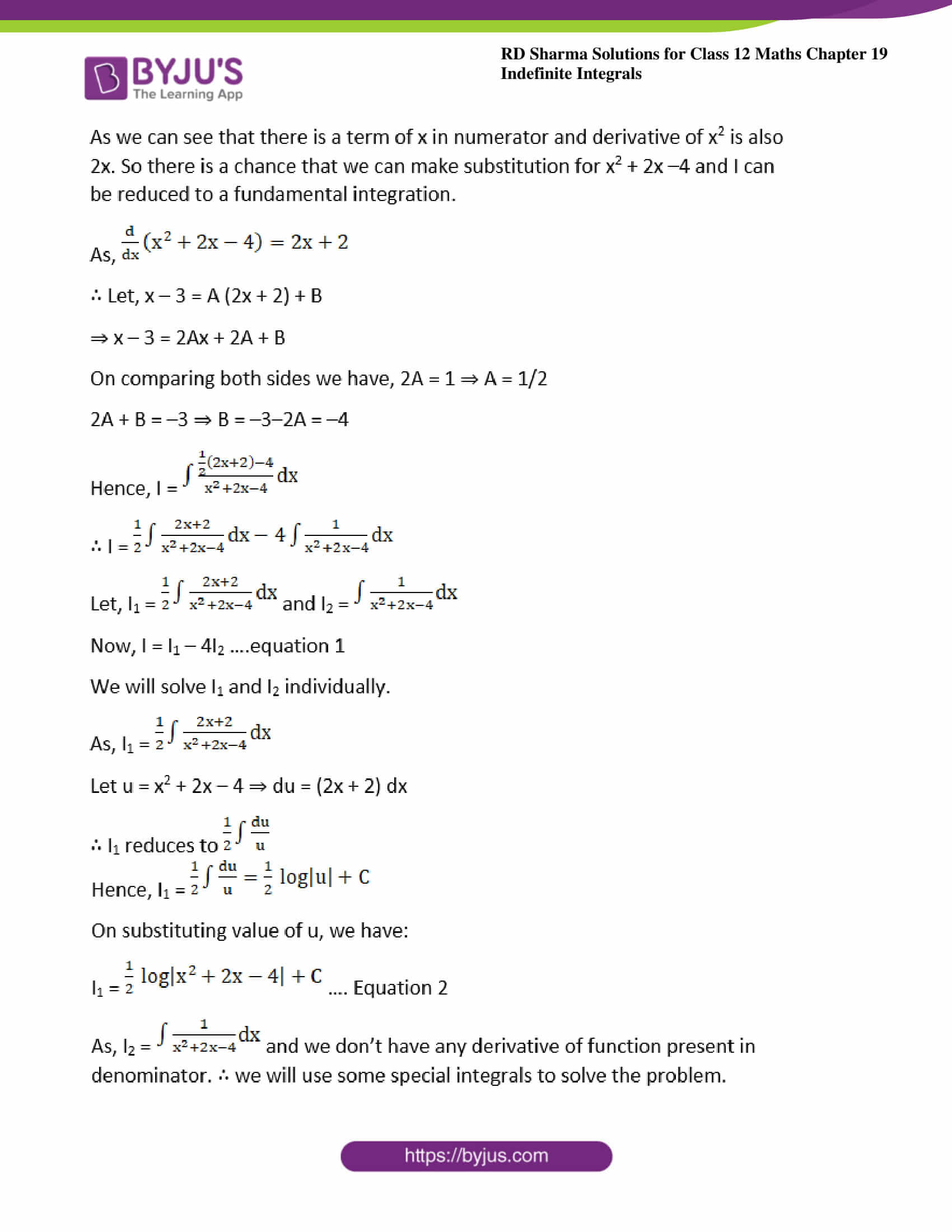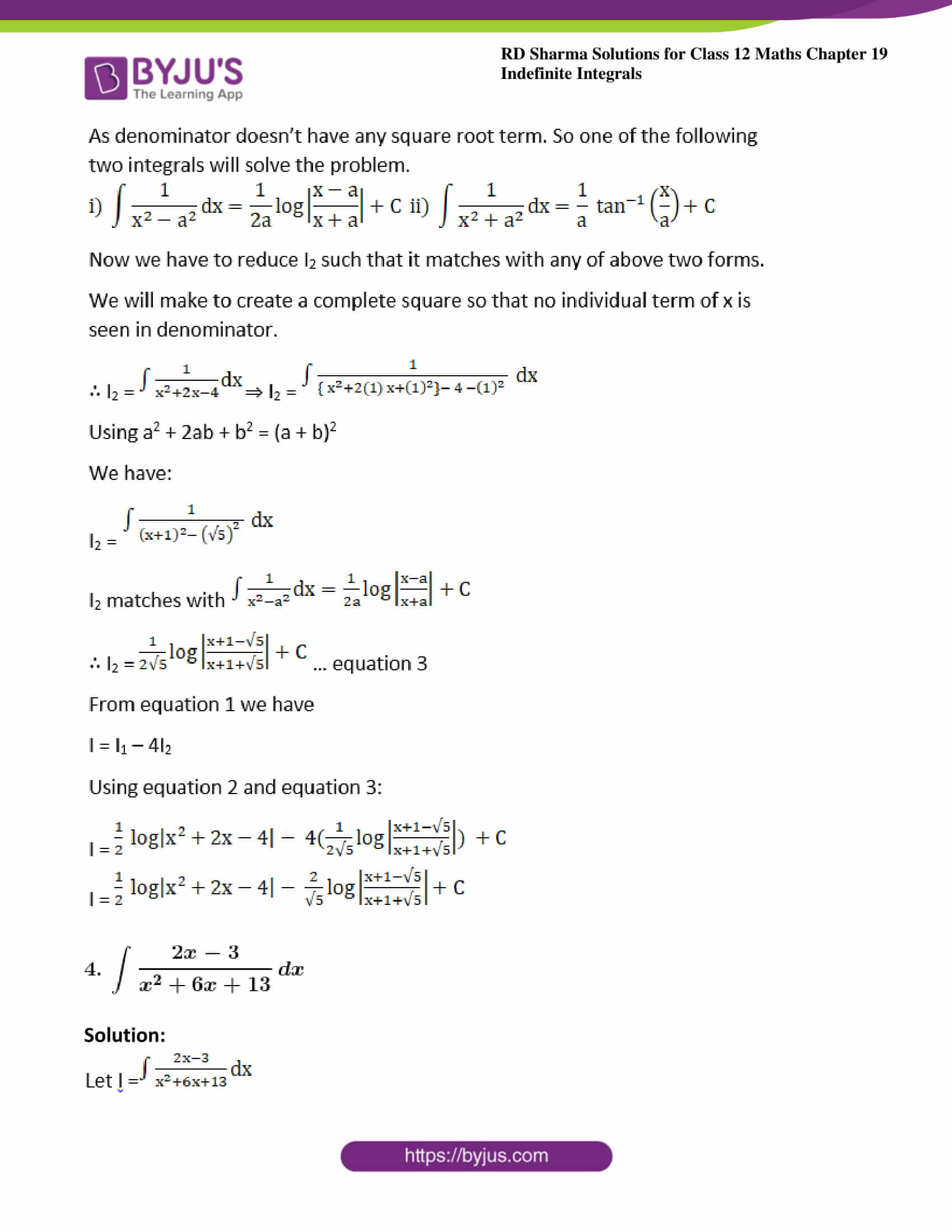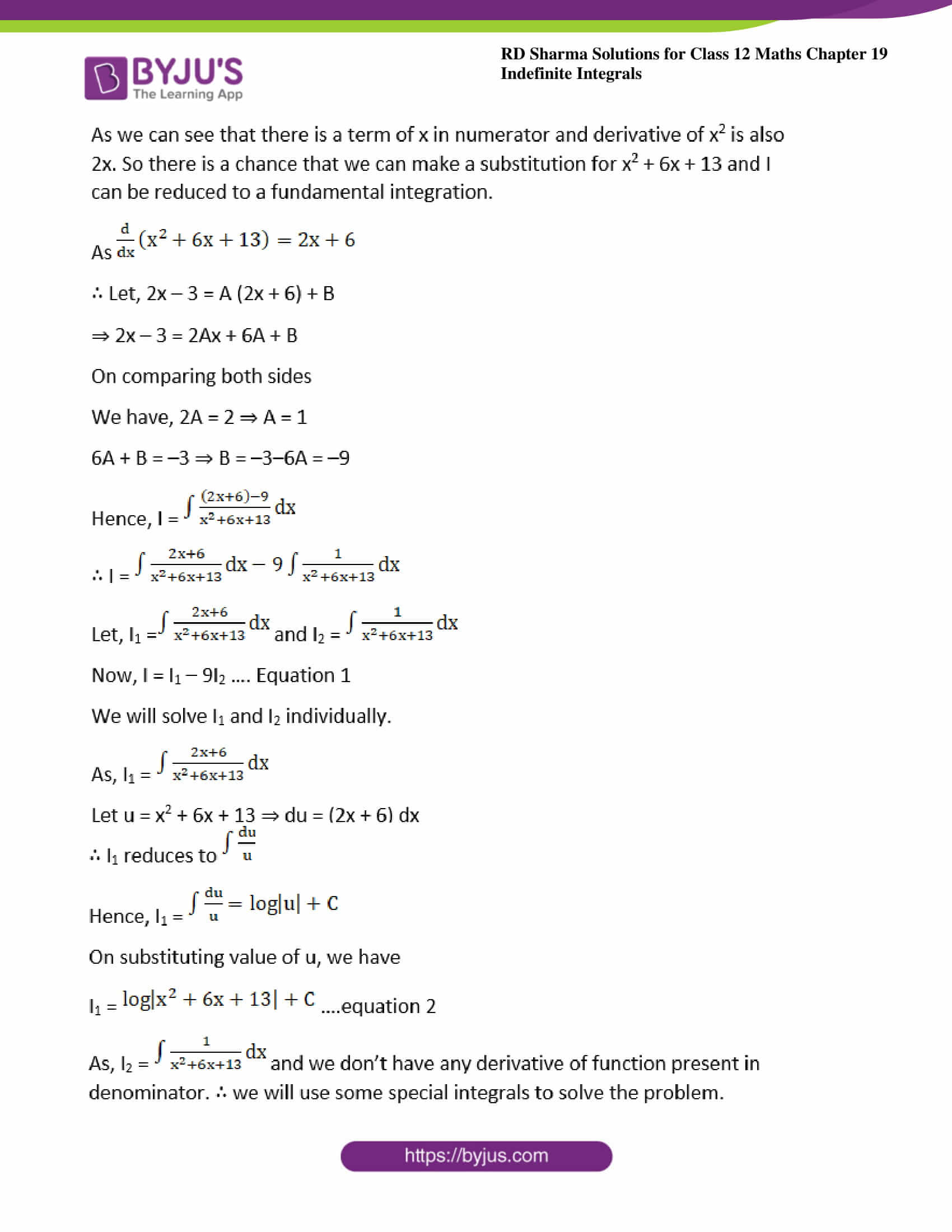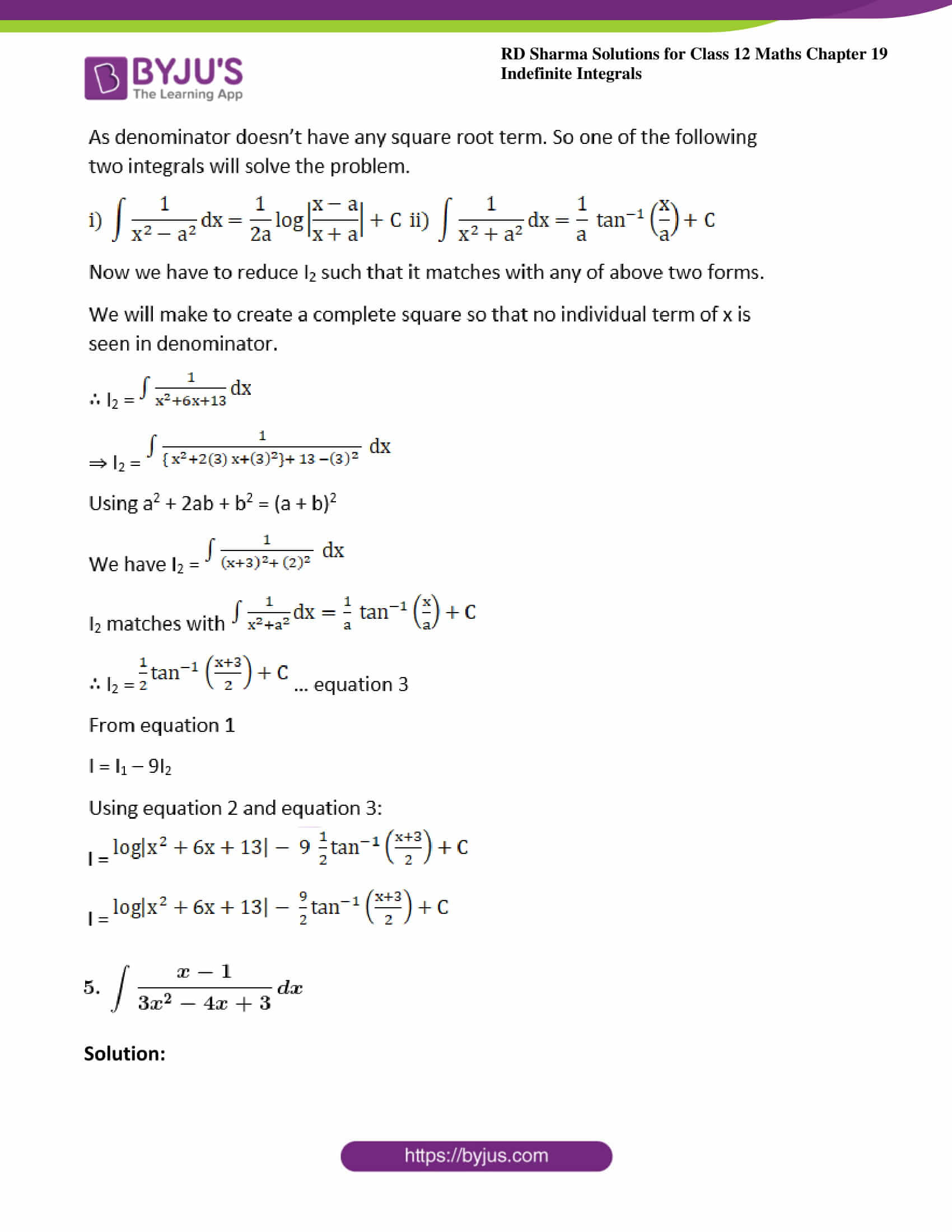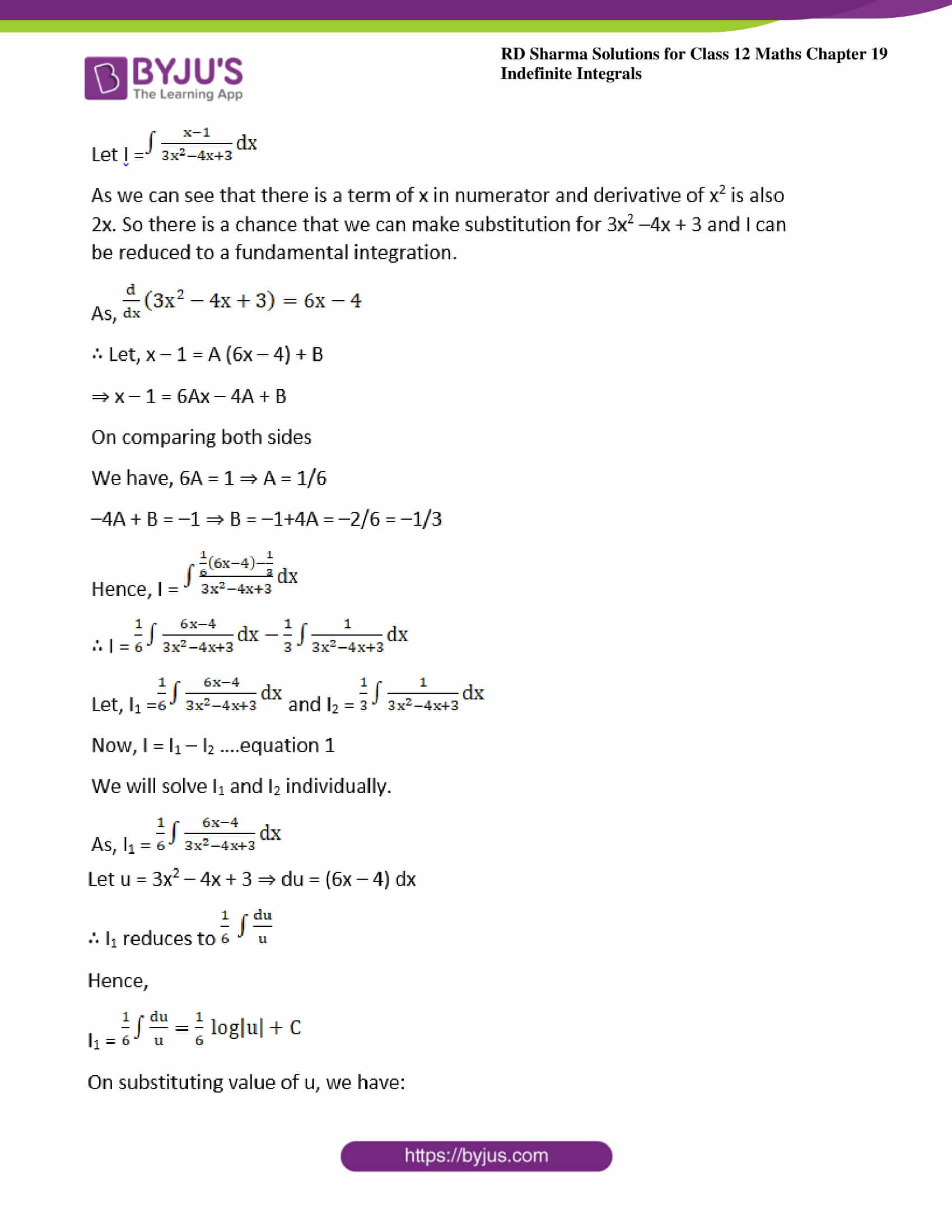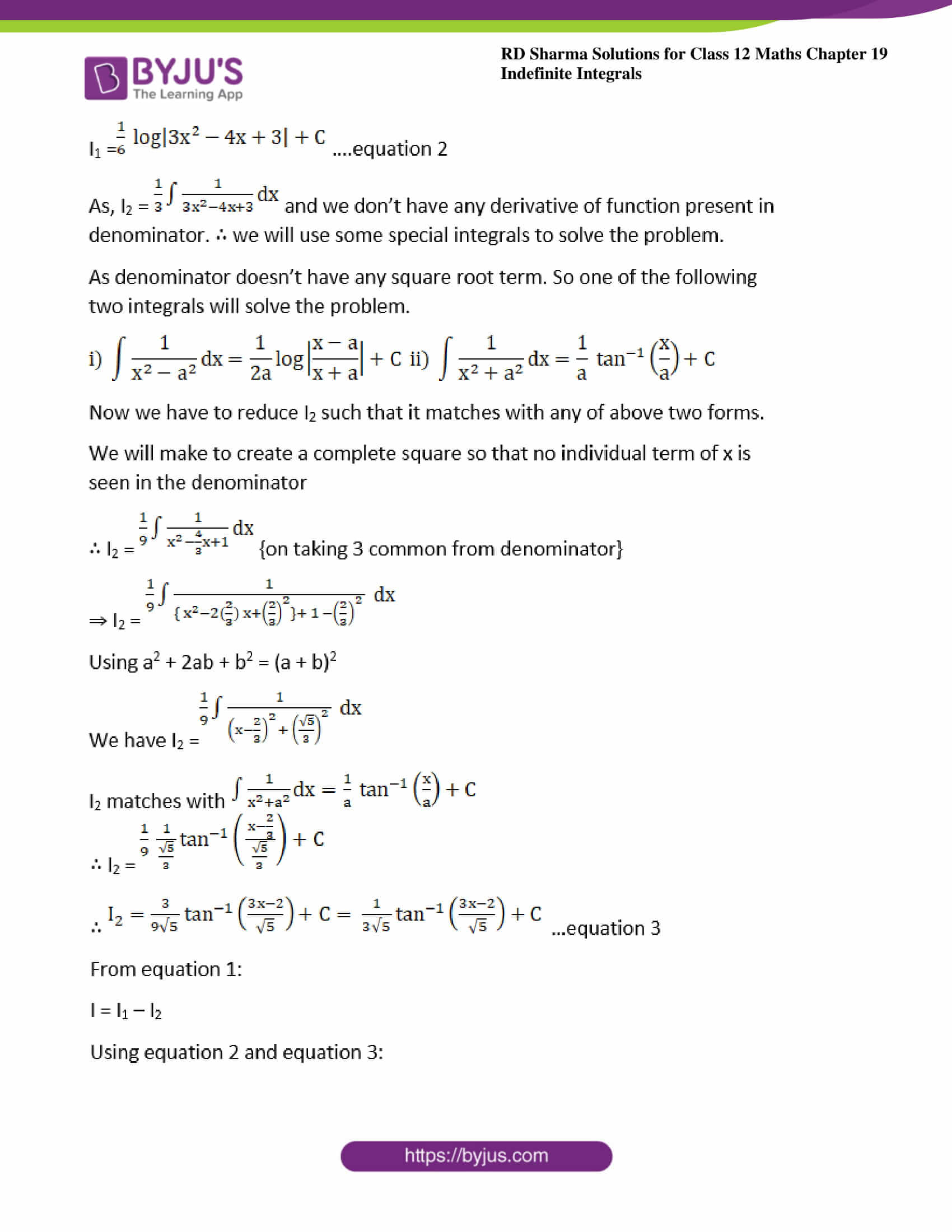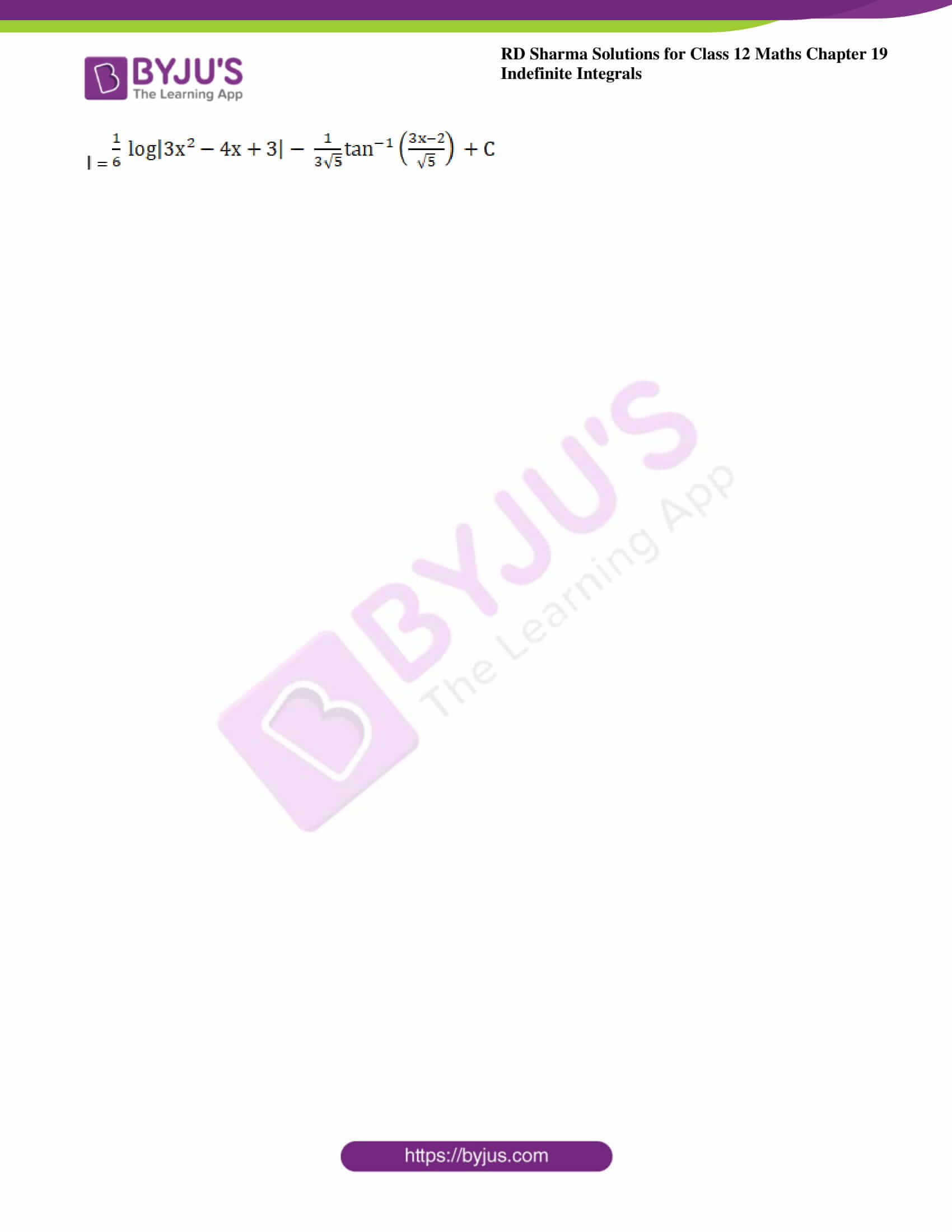### Access other exercises of RD Sharma Solutions For Class 12 Chapter 19 – Indefinite Integrals

Exercise 19.1 Solutions

Exercise 19.2 Solutions

Exercise 19.3 Solutions

Exercise 19.4 Solutions

Exercise 19.5 Solutions

Exercise 19.6 Solutions

Exercise 19.7 Solutions

Exercise 19.8 Solutions

Exercise 19.9 Solutions

Exercise 19.10 Solutions

Exercise 19.11 Solutions

Exercise 19.12 Solutions

Exercise 19.13 Solutions

Exercise 19.14 Solutions

Exercise 19.15 Solutions

Exercise 19.16 Solutions

Exercise 19.17 Solutions

Exercise 19.18 Solutions

Exercise 19.20 Solutions

Exercise 19.21 Solutions

Exercise 19.22 Solutions

Exercise 19.23 Solutions

Exercise 19.24 Solutions

Exercise 19.25 Solutions

Exercise 19.26 Solutions

Exercise 19.27 Solutions

Exercise 19.28 Solutions

Exercise 19.29 Solutions

Exercise 19.30 Solutions

Exercise 19.31 Solutions

Exercise 19.32 Solutions

### Exercise 19.19 Page No: 19.104

Evaluate the following integrals: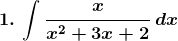Solution: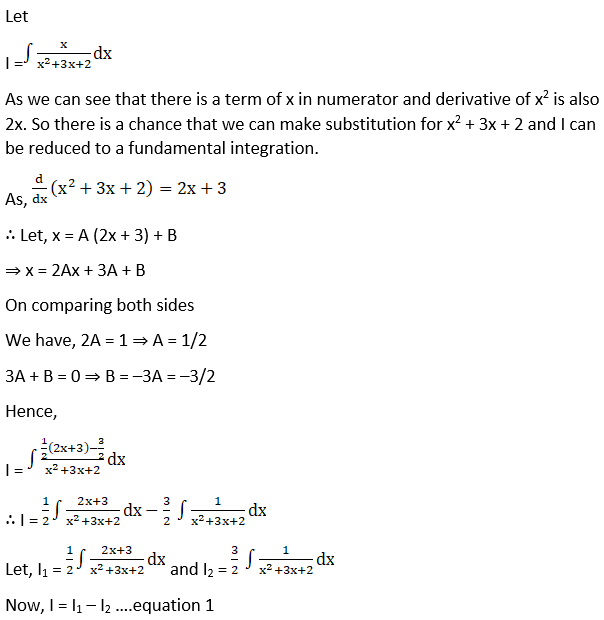We will solve I1 and I2 individually.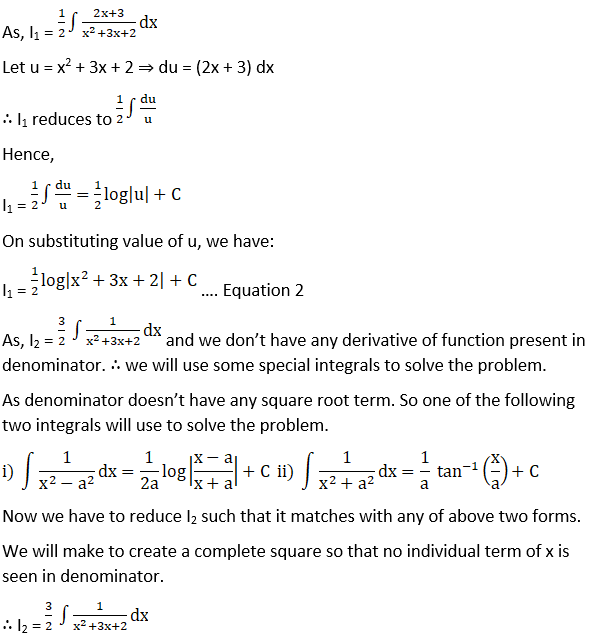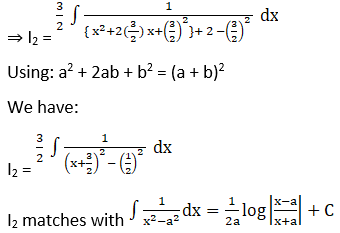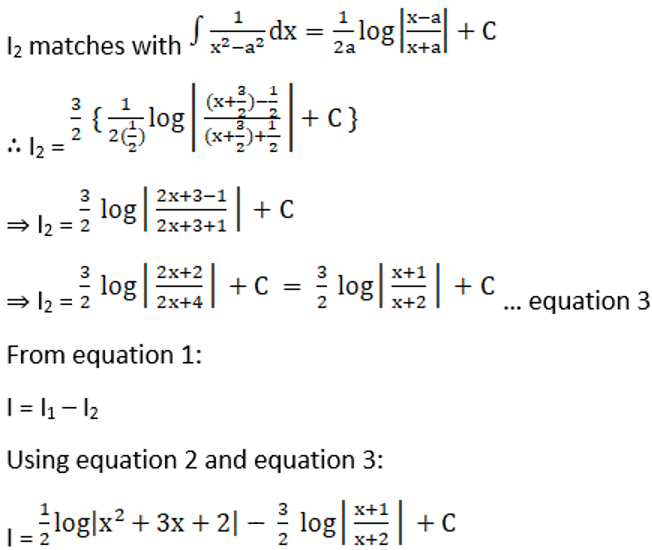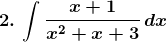Solution: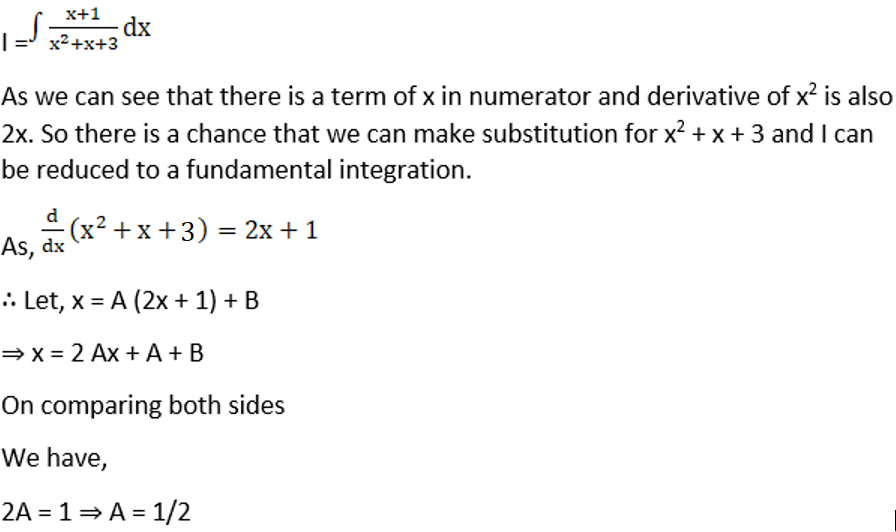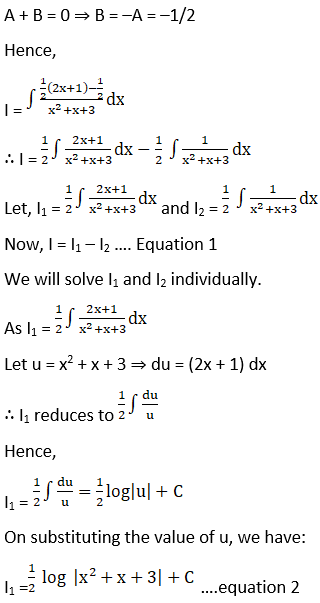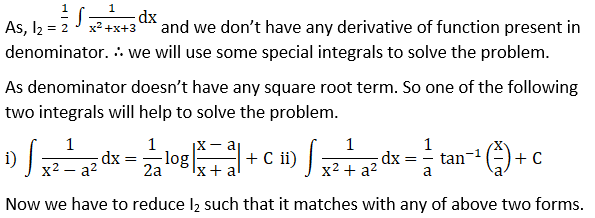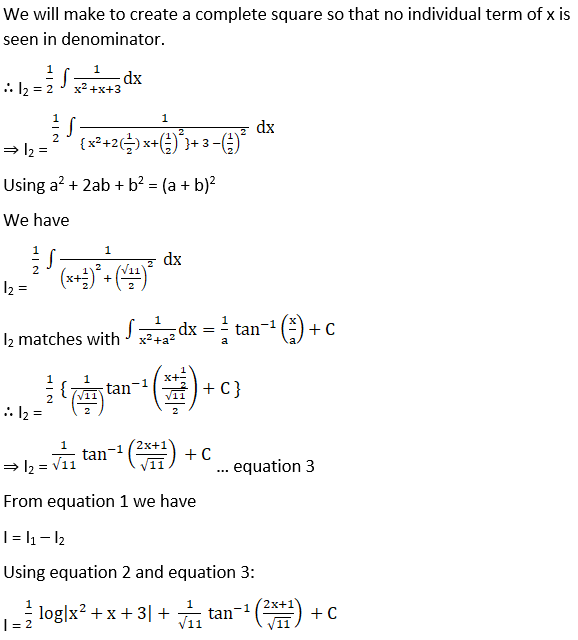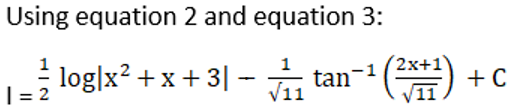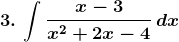Solution: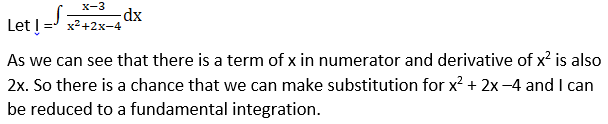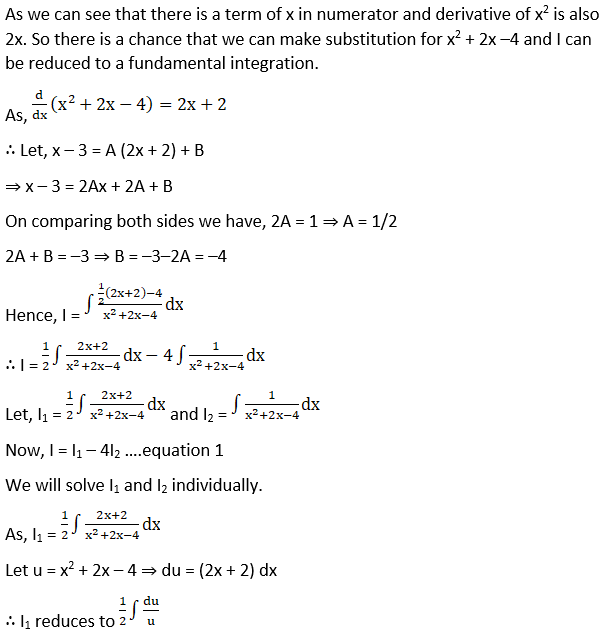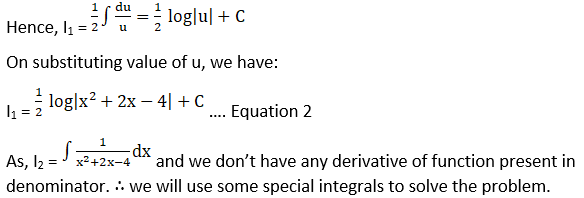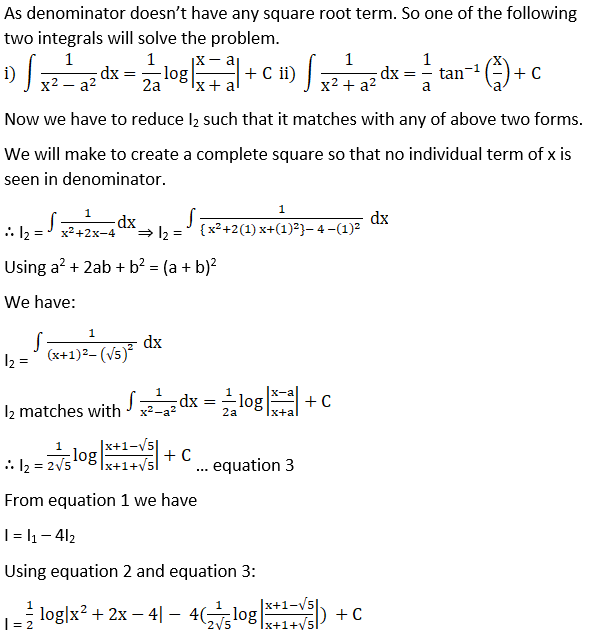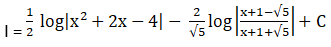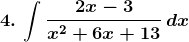Solution: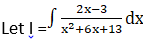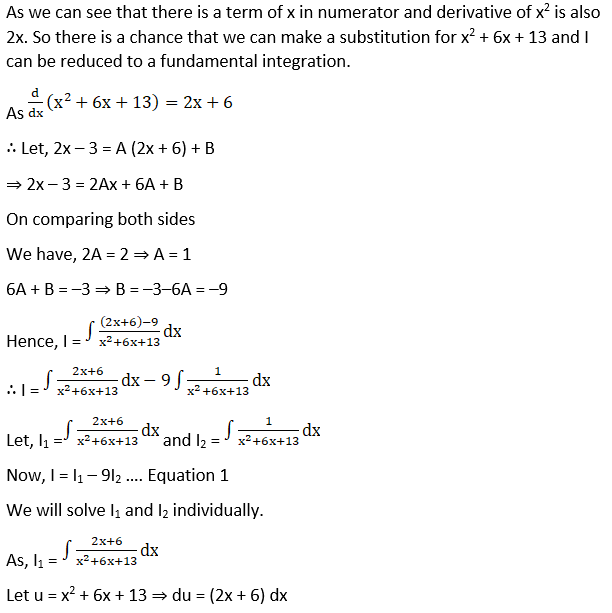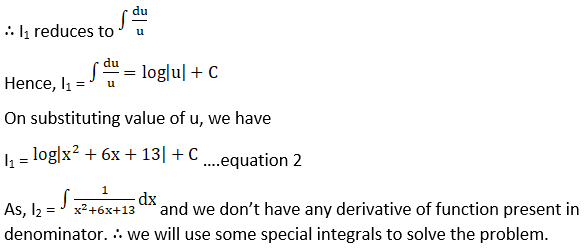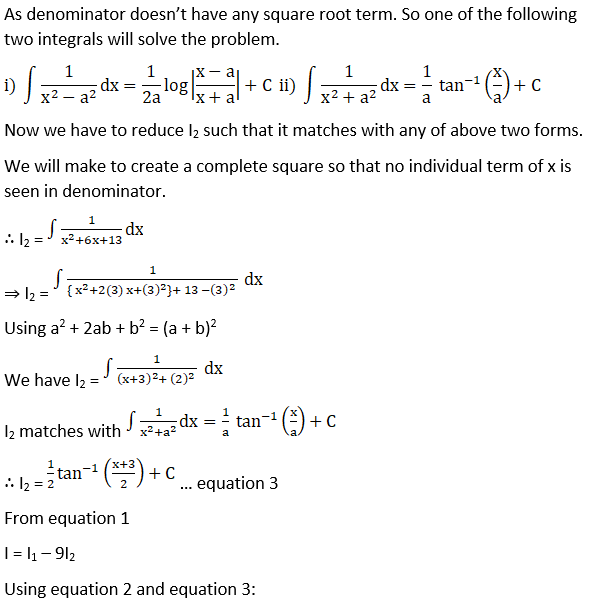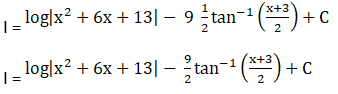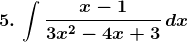Solution: